# Calculator addition of three mixed numbers

The calculator performs basic and advanced operations with mixed numbers, fractions, integers, decimals. Mixed fractions are also called mixed numbers. A mixed fraction is a whole number and a proper fraction combined, i.e. one and three-quarters. The calculator evaluates the expression or solves the equation with step-by-step calculation progress information. Solve problems with two or more mixed numbers fractions in one expression.

### 13/8 + 611/13 + 57/8 = 733/52 = 14 5/52 ≅ 14.0961538

Spelled result in words is fourteen and five fifty-seconds (or seven hundred thirty-three fifty-seconds).

### Calculation steps

1. Conversion a mixed number 1 3/8 to a improper fraction: 1 3/8 = 1 3/8 = 1 · 8 + 3/8 = 8 + 3/8 = 11/8

To find a new numerator:
a) Multiply the whole number 1 by the denominator 8. Whole number 1 equally 1 * 8/8 = 8/8
b) Add the answer from previous step 8 to the numerator 3. New numerator is 8 + 3 = 11
c) Write a previous answer (new numerator 11) over the denominator 8.

One and three eighths is eleven eighths
2. Conversion a mixed number 6 11/13 to a improper fraction: 6 11/13 = 6 11/13 = 6 · 13 + 11/13 = 78 + 11/13 = 89/13

To find a new numerator:
a) Multiply the whole number 6 by the denominator 13. Whole number 6 equally 6 * 13/13 = 78/13
b) Add the answer from previous step 78 to the numerator 11. New numerator is 78 + 11 = 89
c) Write a previous answer (new numerator 89) over the denominator 13.

Six and eleven thirteenths is eighty-nine thirteenths
3. Add: 11/8 + 89/13 = 11 · 13/8 · 13 + 89 · 8/13 · 8 = 143/104 + 712/104 = 143 + 712/104 = 855/104
For adding, subtracting, and comparing fractions, it is suitable to adjust both fractions to a common (equal, identical) denominator. The common denominator you can calculate as the least common multiple of both denominators - LCM(8, 13) = 104. In practice, it is enough to find the common denominator (not necessarily the lowest) by multiplying the denominators: 8 × 13 = 104. In the following intermediate step, the fraction result cannot be further simplified by canceling.
In other words - eleven eighths plus eighty-nine thirteenths = eight hundred fifty-five one-hundred fourths.
4. Conversion a mixed number 5 7/8 to a improper fraction: 5 7/8 = 5 7/8 = 5 · 8 + 7/8 = 40 + 7/8 = 47/8

To find a new numerator:
a) Multiply the whole number 5 by the denominator 8. Whole number 5 equally 5 * 8/8 = 40/8
b) Add the answer from previous step 40 to the numerator 7. New numerator is 40 + 7 = 47
c) Write a previous answer (new numerator 47) over the denominator 8.

Five and seven eighths is forty-seven eighths
5. Add: the result of step No. 3 + 47/8 = 855/104 + 47/8 = 855/104 + 47 · 13/8 · 13 = 855/104 + 611/104 = 855 + 611/104 = 1466/104 = 2 · 733/2 · 52 = 733/52
For adding, subtracting, and comparing fractions, it is suitable to adjust both fractions to a common (equal, identical) denominator. The common denominator you can calculate as the least common multiple of both denominators - LCM(104, 8) = 104. In practice, it is enough to find the common denominator (not necessarily the lowest) by multiplying the denominators: 104 × 8 = 832. In the following intermediate step, cancel by a common factor of 2 gives 733/52.
In other words - eight hundred fifty-five one-hundred fourths plus forty-seven eighths = seven hundred thirty-three fifty-seconds.

## Mixed fractions in word problems:

• A science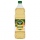A science experiment calls for making 3 and 2/3 cups of distilled water with 1 and 3/5 cups of vinegar and 2/3 cups of liquid detergent. How much liquid in all and cups is needed?Add this two mixed numbers: 1 5/6 + 2 2/11=
• A more than bWhat is 5 1/7 more than 2 3/7?
• Donuts 3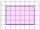You pick up donuts for a party. You have 3 and 4/12 boxes of donuts. Roy picks up donuts too. He has 2 and 9/12 boxes of donuts. How many boxes of donuts do you have in all?
• Sum of 18Sum of two fractions is 4 3/7. If one of the fractions is 2 1/5 find the other one .
• Sum of mixedThe sum of two mixed numbers is 15½. One of the number is 5 ¾, what is the other?
• Dog food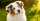A whole bag of dog food weighs 5 1/2 pounds. How much dog food do you have if there are 3 3/4 bags in the cupboard?
• Sum of 20Sum of the two numbers is 15 1/6. If one of the numbers is 2 2/3, find the other number.
• Zailene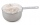Zailene wants to make two kinds of kakanin, puto and kutsina. Puto recipe needs 3 2/5 cup of flour while Kutsinta needs 5 ¼ cup of flour. How many cups of flour will Zailene be needed in all?3 3/4 + 2 3/5 + 5 1/2 Show your solution.Add this two mixed numbers: 1 5/6 + 2 2/11=The first team member in a 926-person relay race must run 2 1/4 laps, the second team member must run 1 1/2 laps, and the third team member must run 3 1/4 laps. How many laps in all must each team run?Add two mixed fractions: 2 4/6 + 1 3/62 and 1/8th plus 1 and 1/3rd =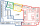Jack planted 1 1/4 rows of lettuce in the family garden, Rose planted 1 1/2 rows of broccoli and Calvin planted 4 5/12 rows of beans. How many rows were planted in the garden?A textile store sold a bolt of denim (what jeans are made out of). In one day, the following number of yards were purchased from the one bolt: 5 2/3, 7, 4 2/3, 8 5/8, 9 3/5, 10 ½, and 8. How many yards were sold?The weight of three packets are 3 2/5 kg + 2 1/3 kg + 5 1/2 kg. Find total weight of all the three packets.